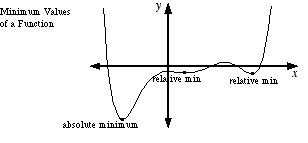index: click on a letter A B C D E F G H I J K L M N O P Q R S T U V W X Y Z A to Z index index: subject areas numbers & symbols sets, logic, proofs geometry algebra trigonometry advanced algebra & pre-calculus calculus advanced topics probability & statistics real world applications multimedia entrieswww.mathwords.com about mathwords website feedback

 Absolute Minimum, Absolute Min Global Minimum, Golbal Min The lowest point over the entire domain of a function or relation. Note: The first derivative test and the second derivative test are common methods used to find minimum values of a function.See also Relative minimum, local minimum, relative maximum, local maximum, absolute maximum, global maximum, extremum